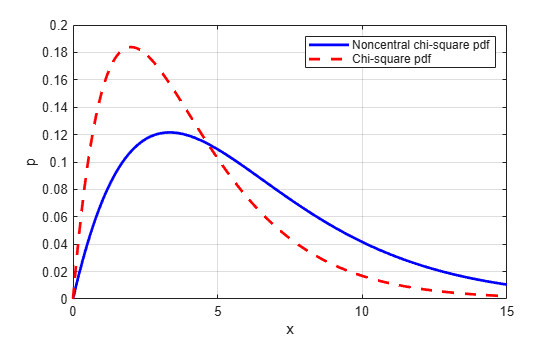# ncx2pdf

Noncentral chi-square probability density function

## Syntax

```Y = ncx2pdf(X,V,DELTA) ```

## Description

`Y = ncx2pdf(X,V,DELTA)` computes the noncentral chi-square pdf at each of the values in `X` using the corresponding degrees of freedom in `V` and positive noncentrality parameters in `DELTA`. Vector or matrix inputs for `X`, `V`, and `DELTA` must have the same size, which is also the size of `Y`. A scalar input for `X`, `V`, or `DELTA` is expanded to a constant array with the same dimensions as the other inputs.

Some texts refer to this distribution as the generalized Rayleigh, Rayleigh-Rice, or Rice distribution.

## Examples

collapse all

Compute the pdf of a noncentral chi-square distribution with degrees of freedom `V = 4` and noncentrality parameter `DELTA = 2`. For comparison, also compute the pdf of a chi-square distribution with the same degrees of freedom.

```x = (0:0.1:10)'; ncx2 = ncx2pdf(x,4,2); chi2 = chi2pdf(x,4);```

Plot the pdf of the noncentral chi-square distribution on the same figure as the pdf of the chi-square distribution.

```figure; plot(x,ncx2,'b-','LineWidth',2) hold on plot(x,chi2,'g--','LineWidth',2) legend('ncx2','chi2')```## References

 Johnson, N., and S. Kotz. Distributions in Statistics: Continuous Univariate Distributions-2. Hoboken, NJ: John Wiley & Sons, Inc., 1970, pp. 130–148.

## Version History

Introduced before R2006a